Back-end

# Write Tests That Matter: Tackle The Most Complex Code First

A CS PhD with over a decade of Java experience, Andrey's a skilled business architect who's always looking to use his math/algorithm skills.
Read the Spanish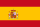version of this article translated by Marisela Ordaz

There are a lot of discussions, articles, and blogs around the topic of code quality. People say - use Test Driven techniques! Tests are a “must have” to start any refactoring! That’s all cool, but it’s 2016 and there is a massive volume of products and code bases still in production that were created ten, fifteen, or even twenty years ago. It’s no secret that a lot of them have legacy code with low test coverage.

While I’d like to be always at the leading, or even bleeding, edge of the technology world - engaged with new cool projects and technologies – unfortunately it’s not always possible and often I have to deal with old systems. I like to say that when you develop from scratch, you act as a creator, mastering new matter. But when you’re working on legacy code, you’re more like a surgeon – you know how the system works in general, but you never know for sure whether the patient will survive your “operation”. And since it’s legacy code, there are not many up to date tests for you to rely on. This means that very frequently one of the very first steps is to cover it with tests. More precisely, not merely to provide coverage, but to develop a test coverage strategy.Forget 100% coverage. Test smarter by identifying classes that are more likely to break.

Basically, what I needed to determine was what parts (classes / packages) of the system we needed to cover with tests in the first place, where we needed unit tests, where integration tests would be more helpful etc. There are admittedly many ways to approach this type of analysis and the one that I’ve used may not be the best, but it’s kind of an automatic approach. Once my approach is implemented, it takes minimal time to actually do the analysis itself and, what is more important, it brings some fun into legacy code analysis.

The main idea here is to analyse two metrics – coupling (i.e., afferent coupling, or CA) and complexity (i.e. cyclomatic complexity).

The first one measures how many classes use our class, so it basically tells us how close a particular class is to the heart of the system; the more classes there are that use our class, the more important it is to cover it with tests.

On the other hand, if a class is very simple (e.g. contains only constants), then even if it’s used by many other parts of the system, it’s not nearly as important to create a test for. Here is where the second metric can help. If a class contains a lot of logic, the Cyclomatic complexity will be high.

The same logic can also be applied in reverse; i.e., even if a class is not used by many classes and represents just one particular use case, it still makes sense to cover it with tests if its internal logic is complex.

There is one caveat though: let’s say we have two classes – one with the CA 100 and complexity 2 and the other one with the CA 60 and complexity 20. Even though the sum of the metrics is higher for the first one we should definitely cover the second one first. This is because the first class is being used by a lot of other classes, but is not very complex. On the other hand, the second class is also being used by a lot of other classes but is relatively more complex than the first class.

To summarize: we need to identify classes with high CA and Cyclomatic complexity. In mathematical terms, a fitness function is needed that can be used as a rating - f(CA,Complexity) - whose values increase along with CA and Complexity.

Generally speaking, the classes with the smallest differences between the two metrics should be given the highest priority for test coverage.

Finding tools to calculate CA and Complexity for the whole code base, and provide a simple way to extract this information in CSV format, proved to be a challenge. During my search, I came across two tools that are free so it would be unfair not to mention them:

## A Bit Of Math

The main problem here is that we have two criteria – CA and Cyclomatic complexity – so we need to combine them and convert into one scalar value. If we had a slightly different task – e.g., to find a class with the worst combination of our criteria – we would have a classical multi-objective optimization problem: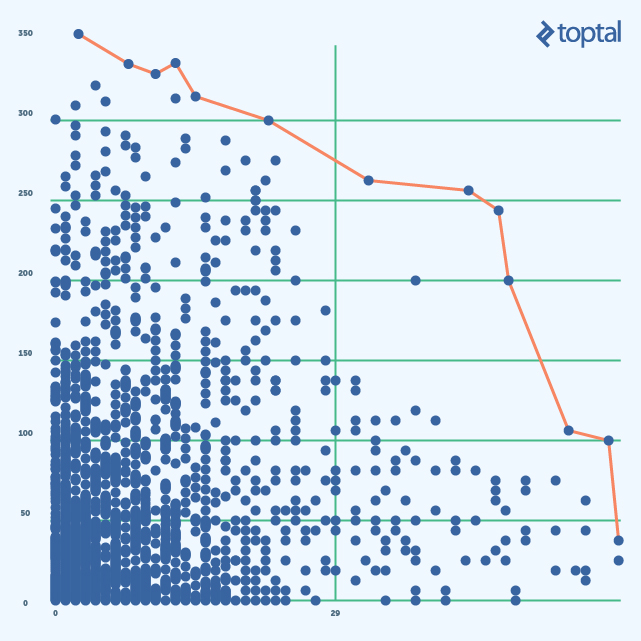We would need to find a point on the so called Pareto front (red in the picture above). What is interesting about the Pareto set is that every point in the set is a solution to the optimization task. Whenever we move along the red line we need to make a compromise between our criteria – if one gets better the other one gets worse. This is called Scalarization and the final result depends on how we do it.

There are a lot of techniques that we can use here. Each has its own pros and cons. However, the most popular ones are linear scalarizing and the one based on an reference point. Linear is the easiest one. Our fitness function will look like a linear combination of CA and Complexity:

f(CA, Complexity) = A×CA + B×Complexity

where A and B are some coefficients.

The point which represents a solution to our optimization problem will lie on the line (blue in the picture below). More precisely, it will be at the intersection of the blue line and red Pareto front. Our original problem is not exactly an optimization problem. Rather, we need to create a ranking function. Let’s consider two values of our ranking function, basically two values in our Rank column:

R1 = A∗CA + B∗Complexity and R2 = A∗CA + B∗Complexity

Both of the formulas written above are equations of lines, moreover these lines are parallel. Taking more rank values into consideration we’ll get more lines and therefore more points where the Pareto line intersects with the (dotted) blue lines. These points will be classes corresponding to a particular rank value.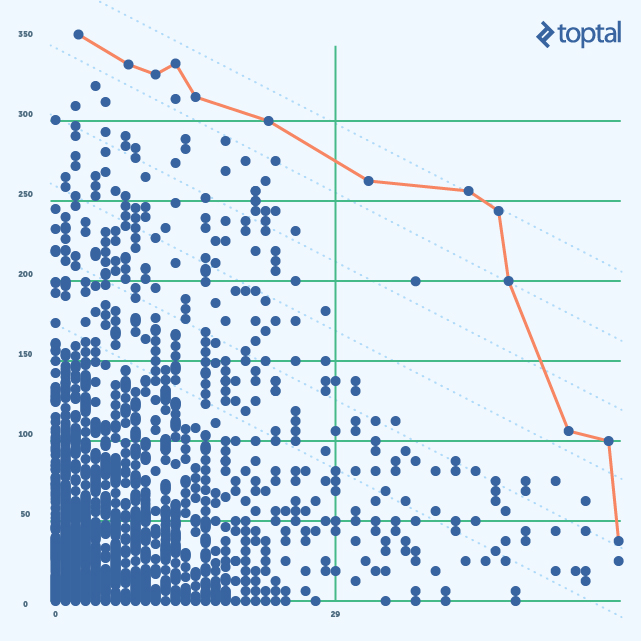Unfortunately, there is an issue with this approach. For any line (Rank value), we’ll have points with very small CA and very big Complexity (and visa versa) lying on it. This immediately puts points with a big difference between metric values in the top of the list which is exactly what we wanted to avoid.

The other way to do the scalarizing is based on the reference point. Reference point is a point with the maximum values of both criteria:

(max(CA), max(Complexity))

The fitness function will be the distance between the Reference point and the data points:

f(CA,Complexity) = √((CA−CA )2 + (Complexity−Complexity)2)

We can think about this fitness function as a circle with the center at the reference point. The radius in this case is the value of the Rank. The solution to the optimization problem will be the point where the circle touches the Pareto front. The solution to the original problem will be sets of points corresponding to the different circle radii as shown in the following picture (parts of circles for different ranks are shown as dotted blue curves):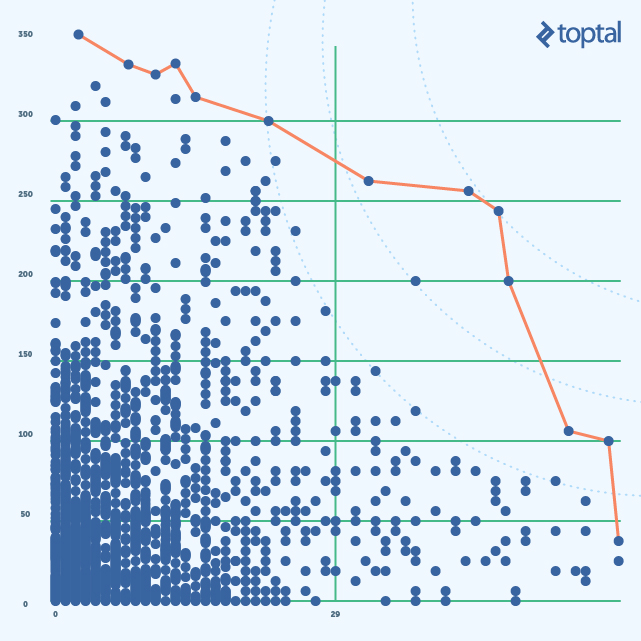This approach deals better with extreme values but there are still two issues: First – I’d like to have more points near the reference points to better overcome the problem that we’ve faced with linear combination. Second – CA and Cyclomatic complexity are inherently different and have different values set, so we need to normalize them (e.g. so that all the values of both metrics would be from 1 to 100).

Here is a small trick that we can apply to solve the first issue – instead of looking at the CA and Cyclomatic Complexity, we can look at their inverted values. The reference point in this case will be (0,0). To solve the second issue, we can just normalize metrics using minimum value. Here is how it looks:

Inverted and normalized complexity – NormComplexity:

(1 + min(Complexity)) / (1 + Complexity)∗100

Inverted and normalized CA – NormCA:

(1 + min(CA)) / (1+CA)∗100

Note: I added 1 to make sure that there is no division by 0.

The following picture shows a plot with the inverted values: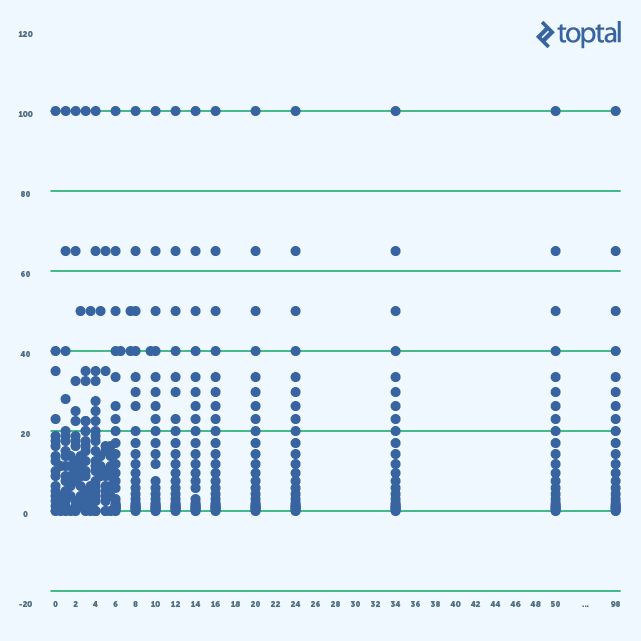## Final Ranking

We are now coming to the last step - calculating the rank. As mentioned, I’m using the reference point method, so the only thing that we need to do is to calculate the length of the vector, normalize it, and make it ascend with the importance of a unit test creation for a class. Here is the final formula:

Rank(NormComplexity , NormCA) = 100 − √(NormComplexity2 + NormCA2) / √2

## More Statistics

There is one more thought that I’d like to add, but let’s first have a look at some statistics. Here is a histogram of the Coupling metrics: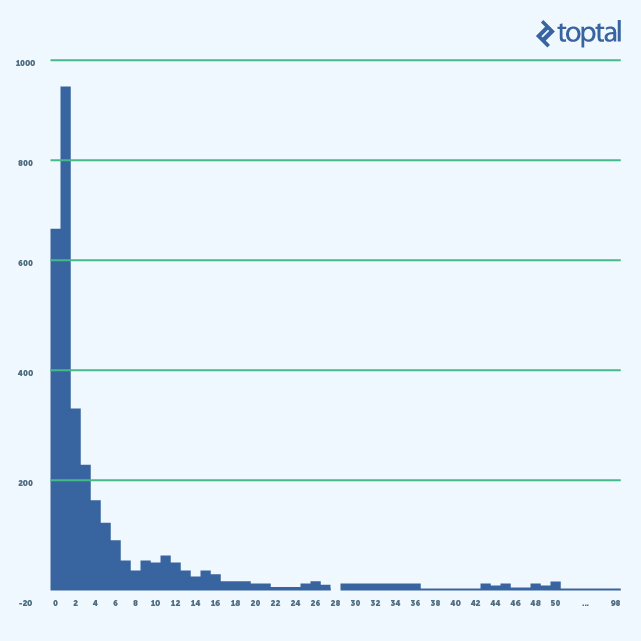What is interesting about this picture is the number of classes with low CA (0-2). Classes with CA 0 are either not used at all or are top level services. These represent API endpoints, so it’s fine that we have a lot of them. But classes with CA 1 are the ones that are directly used by the endpoints and we have more of these classes than endpoints. What does this mean from architecture / design perspective?

In general, it means that we have a kind of script oriented approach – we script every business case separately (we can’t really reuse the code as business cases are too diverse). If that is the case, then it’s definitely a code smell and we need to do refactoring. Otherwise, it means the cohesion of our system is low, in which case we also need refactoring, but architectural refactoring this time.

Additional useful information we can get from the histogram above is that we can completely filter out classes with low coupling (CA in {0,1}) from the list of the classes eligible for coverage with unit tests. The same classes, though, are good candidates for the integration / functional tests.

You can find all the scripts and resources that I have used in this GitHub repository: ashalitkin/code-base-stats.

## Does It Always Work?

Not necessarily. First of all it’s all about static analysis, not runtime. If a class is linked from many other classes it can be a sign that it’s heavily used, but it’s not always true. For example, we don’t know whether the functionality is really heavily used by end users. Second, if the design and the quality of the system is good enough, then most likely different parts / layers of it are decoupled via interfaces so static analysis of the CA will not give us a true picture. I guess it’s one of the main reasons why CA is not that popular in tools like Sonar. Fortunately, it’s totally fine for us since, if you remember, we are interested in applying this specifically to old ugly code bases.

In general, I’d say that runtime analysis would give much better results, but unfortunately it’s much more costly, time consuming, and complex, so our approach is a potentially useful and lower cost alternative.